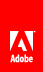# Using Matrix objects

Flash Player 9 and later, Adobe AIR 1.0 and later

 The Matrix class represents a transformation matrix that determines how to map points from one coordinate space to another. You can perform various graphical transformations on a display object by setting the properties of a Matrix object, applying that Matrix object to the matrix property of a Transform object, and then applying that Transform object as the transform property of the display object. These transformation functions include translation ( x and y repositioning), rotation, scaling, and skewing. Although you could define a matrix by directly adjusting the properties ( a , b , c , d , tx , ty ) of a Matrix object, it is easier to use the createBox() method. This method includes parameters that let you directly define the scaling, rotation, and translation effects of the resulting matrix. For example, the following code creates a Matrix object that scales an object horizontally by 2.0, scales it vertically by 3.0, rotates it by 45°, moving (translating) it 10 pixels to the right, and moving it 20 pixels down: ```var matrix:Matrix = new Matrix(); var scaleX:Number = 2.0; var scaleY:Number = 3.0; var rotation:Number = 2 * Math.PI * (45 / 360); var tx:Number = 10; var ty:Number = 20; matrix.createBox(scaleX, scaleY, rotation, tx, ty);``` You can also adjust the scaling, rotation, and translation effects of a Matrix object by using the scale() , rotate() , and translate() methods. Note that these methods combine with the values of the existing Matrix object. For example, the following code sets a Matrix object that scales an object by a factor of 4 and rotates it 60°, since the scale() and rotate() methods are called twice: ```var matrix:Matrix = new Matrix(); var rotation:Number = 2 * Math.PI * (30 / 360); // 30° var scaleFactor:Number = 2; matrix.scale(scaleFactor, scaleFactor); matrix.rotate(rotation); matrix.scale(scaleX, scaleY); matrix.rotate(rotation); myDisplayObject.transform.matrix = matrix;``` To apply a skew transformation to a Matrix object, adjust its b or c property. Adjusting the b property skews the matrix vertically, and adjusting the c property skews the matrix horizontally. The following code skews the myMatrix Matrix object vertically by a factor of 2: ```var skewMatrix:Matrix = new Matrix(); skewMatrix.b = Math.tan(2); myMatrix.concat(skewMatrix);``` You can apply a Matrix transformation to the transform property of a display object. For example, the following code applies a matrix transformation to a display object named myDisplayObject : ```var matrix:Matrix = myDisplayObject.transform.matrix; var scaleFactor:Number = 2; var rotation:Number = 2 * Math.PI * (60 / 360); // 60° matrix.scale(scaleFactor, scaleFactor); matrix.rotate(rotation); myDisplayObject.transform.matrix = matrix;``` The first line sets a Matrix object to the existing transformation matrix used by the myDisplayObject display object (the matrix property of the transformation property of the myDisplayObject display object). This way, the Matrix class methods that you call have a cumulative effect on the display object’s existing position, scale, and rotation. Note: The ColorTransform class is also included in the flash.geometry package. This class is used to set the colorTransform property of a Transform object. Since it does not apply any geometrical transformation, it is not discussed, in detail, here. For more information, see the ColorTransform class in the ActionScript 3.0 Reference for the Adobe Flash Platform .Twitter™ and Facebook posts are not covered under the terms of Creative Commons.// Ethnio survey code removed